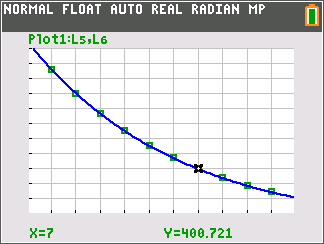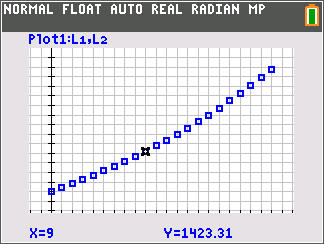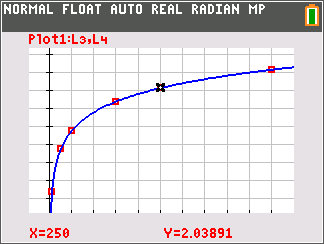# Activities

••• ##### Subject Area

• Math: Algebra II: Logarithms and Exponentials
• Math: Algebra II: Logarithmic Functions

• ##### Author9-12

45 Minutes

• ##### Device
• TI-84 Plus
• TI-84 Plus Silver Edition
•TI-84 Plus C Silver Edition
•TI-84 Plus CE
• ##### Software

TI Connect™ CE

• ##### Report an Issue

Modeling Data#### Activity Overview

Students graph data modeling exponential and logarithmic growth and find equations representing the data.

#### Key Steps

•In Problem 1, students will find the interest rate for given data to determine an interest rate equation. In this problem, they use trial and error to find the equation that models the data.

•In Problem 2, students will determine a logarithmic function that models the given data. In this problem, they use the natural log regression function.